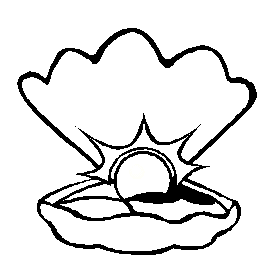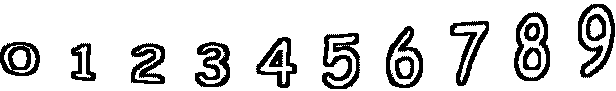4 - 3 =        2 - 0 =3 - 1 =        3 - 0 =        3 - 3 =        2 - 2 = 4 - 0 =        2 - 2 =        0 - 0 =        7 - 5 =